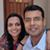D

#### Calculus is hard man

60 viewed last edited 5 years agoAnonymous
1
Question: Find the volume of a solid whose base is the Q1 region bounded by y=√x from [0,9]. Cross sections perpendicular to the x axis are semicircles. Use FTC to solve. I don't know if I should use dy or dx as the bases. Also how do I integrate it when it's squared.Mahesh Godavarti
5

The trick is to recognize in which direction to integrate. The information given shows that you have to integrate along the x-axis. Here is a picture that will help you understand.Essentially volume is given by

\int \int \int dx dy dz

You can either integrate along the x co-ordinate first, y co-ordinate second and then finally across the z co-ordinate.

However, the information is given that each slice perpendicular to the x-axis (e.g. along the y-z plane) is a semi-circle. What I have shown in the picture is a quarter circle.

So, we are already given the information on what the expression inside the parentheses of \int (\int \int dy dz) dx evaluates to.

It evaluates to \frac{1}{2} \pi x (wow!) Why? Because area of a semi-circle is \frac{1}{2} \pi r^2 and the radius of the semi-circle at x is given by \sqrt{x} (this is given to us from the fact that the curve follows the relationship y = \sqrt{x} )

Therefore, the volume evaluates to:

$\int_0^9 \frac{1}{2} \pi x dx = \frac{\pi}{4} [9^2 - 0^2] = \frac{81 \pi}{4}$.

I hope this helps. Ask me if you have any questions.3

Integration is a limit of a sum (called a Riemann sum, not sure if that's in your curriculum, since it's often glossed over). You could set up the sum either way in this case. SInce it's a volume, it becomes a sum of small cylinders (infinitesmal cylinders in the limit)

1) Limit of Sum of (cross sectional area perpendicular to x . delta_x) = Integral (cross sectional area perpendicular to x . dx)

2) Limit of Sum of (cross sectional area perpendicular to y . delta_y) = Integral (cross sectional area perpendicular to y . dy)

Which one do you pick? You pick the one which gives you an easier expression to work with for cross sectional area.

Here, if we take option 1), cross sections perpendicular to x are semicircles with an easy expression for area.

If we go with 2), or the other hand, the cross sectional area is rather complex. You could do that, but that will end up with a proper double integral. If you're in high school, you probably aren't required to know how to do that.

In any case, we can see that 1) is way easier, so we pick that option for elegance and tractability.Mahesh Godavarti
1

Hello, the expression you will have is

\int_0^9 ( \int_0^{\sqrt{x}} f(x, y) dy) dx .

Note that you will be integrating along both x and y.

BTW, I think you forgot to mention that the cross-section is perpendicular to both the axes.

I hope this unblocks you for now. I will post the expression for f(x,y) and how to actually integrate it in the next post.Srinivas Gunturi
1

It usually depends on which is independent and which is dependent in the range that the definite integral is evaluated. In this case, x is in the range [0,9], for every given value of x, there is a circular disc in the [y,z] dimensions with radius $\sqrt[]{x}$. Therefore, the integral will be evaluated on y and z dimensions and finally on the x dimension. And it really doesnt matter whether y is evaluated first or z. Put it down to bias that most prefer to work with x is there is one unknown, (x,y) if 2 unknowns, (x,y,z) if 3 unknowns.

I am attaching the solution here and you can see that even I had the same bias I referred to above :-). I first evaluated on z, then y and finally x.Sindhuja Parimi
0

Sometimes it is easier to use horizontal axis than vertical axis. It also depends on the method you choose to find the volume.

x=f(y) think it is horizontal axis.

y=g(x) think it is vertical axis.

The Integration of square root can be calculated by the formula

\int_{ }^{ }x^n=\frac{x^{n+1}}{n+1}+c

where n=1/2 for square root.

and c is constant.